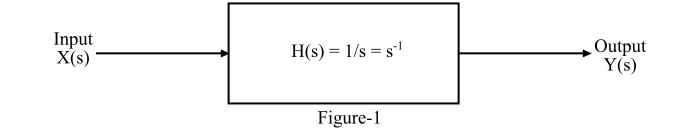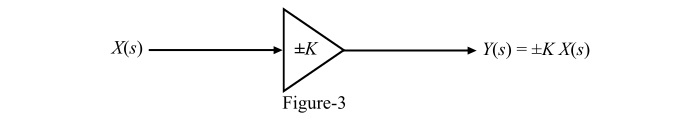# Basic Elements to Construct the Block-Diagram of Continuous-Time Systems

## System Realization

The realization of a continuous-time system means obtaining a network corresponding to the differential equation or transfer function of the system.

## Block Diagram

A diagram of a system in which the main parts or functions are represented by blocks connected by the lines that show the relationship of the blocks is called the block diagram of that system.

## Elements to Construct the Block-Diagram of Continuous- Time System

The transfer function of a continuous-time system can be realised either by using integrators or differentiators. Although, due to certain drawbacks, the differentiators are not used to realise the practical systems. The chief drawback of differentiators is that they amplify the noise signals of high frequency, while the integrators suppress the high frequency noise signals. Therefore, to realise the practical continuous-time systems, only integrators are used. In addition to the integrators and differentiators, the adders and multipliers are also used to construct a block diagram of a continuous-time system.

Hence, the basic elements which are used to construct the block-diagram of a continuous-time system are −

• Integrator

• Multiplier

## Integrator

• The integrator is an element which is used to integrate the input signal of a system.

• The transfer function of an ideal integrator is given by,

$$\mathrm{\mathit{H}\mathrm{\left(\mathit{s}\right)}\:\mathrm{=}\:\frac{1}{\mathit{s}}\:\mathrm{=}\:\mathit{s^{-\mathrm{1}}}}$$

• The main advantage of using integrator in the realization of a continuous-time system is that the integrator suppresses the high frequency noise signals. Because of this reason, only integrators are used to realize the practical continuous-time systems.

• The block diagram representation of an ideal integrator is shown in Figure-1.• The adder is an element which is used to perform the addition and subtraction of the signals.

• It is also called the summer circuit.

• The output of the adder is the algebraic sum of all the input variables.

• Graphically, it is represented by a small circle that has at least two input terminals and one output terminal as shown in Figure-2.## Multiplier

• The multiplier is an element which is used to multiply the input signal by a constant.

• The gain (or constant) of the multiplier can be positive or negative.

• The magnitude of the gain of the multiplier may be greater or less than 1.

• The graphical representation of the multiplier is shown in Figure-3.## When is a continuous-time system not physically realizable?

Consider an nth order continuous-time system represented by the following differential equation −

$$\mathrm{\frac{\mathrm{\mathit{d}} ^{\mathit{n}}}{\mathrm{\mathit{d}}\mathit{t}^{\mathit{n}}}\mathit{y}\mathrm{\left(\mathit{t}\right)}\:\mathrm{+}\:\mathit{a_{n-\mathrm{1}}}\frac{\mathrm{\mathit{d}} ^{\mathit{n-\mathrm{1}}}}{\mathrm{\mathit{d}}t^{\mathit{n-\mathrm{1}}}}\mathit{y}\mathrm{\left(\mathit{t}\right)}\:\mathrm{+}\mathit{a_{n-\mathrm{2}}}\frac{\mathrm{\mathit{d}} ^{\mathit{n-\mathrm{2}}}}{\mathrm{\mathit{d}}t^{\mathit{n-\mathrm{2}}}}\mathit{y}\mathrm{\left(\mathit{t}\right)}\:\mathrm{+}...\:\mathrm{+}\:\mathit{a_{\mathrm{1}}\frac{\mathit{d}}{\mathit{dt}}y\mathrm{\left ( \mathit{t} \right )}}\:\mathrm{+}\:\mathit{a_{\mathrm{0}}}\mathit{y}\mathrm{\left(\mathit{t}\right)}\:\mathrm{=}\:\mathit{b_{m}}\frac{\mathrm{\mathit{d}} ^{\mathit{m}}}{\mathrm{\mathit{d}}\mathit{t}^{\mathit{m}}}\mathit{x}\mathrm{\left(\mathit{t}\right)}\:\mathrm{+}\:\mathit{b_{m-\mathrm{1}}}\frac{\mathrm{\mathit{d}} ^{\mathit{m-\mathrm{1}}}}{\mathrm{\mathit{d}}t^{\mathit{m-\mathrm{1}}}}\mathit{x}\mathrm{\left(\mathit{t}\right)}\:\mathrm{+}\:\mathit{b_{m-\mathrm{2}}}\frac{\mathrm{\mathit{d}} ^{\mathit{m-\mathrm{2}}}}{\mathrm{\mathit{d}}t^{\mathit{m-\mathrm{2}}}}\mathit{x}\mathrm{\left(\mathit{t}\right)}\:\mathrm{+}\:...\:\mathrm{+}\:\mathit{b_{\mathrm{1}}\frac{\mathit{d}}{\mathit{dt}}x\mathrm{\left ( \mathit{t} \right )}}\:\mathrm{+}\:\mathit{b_{\mathrm{0}}}\mathit{x}\mathrm{\left(\mathit{t}\right)}}$$

Taking Laplace transform, we get,

$$\mathrm{\Rightarrow\mathit{s^{n}\:\mathrm{+}\:\mathit{a_{n-\mathrm{1}}s}^{n-\mathrm{1}}\mathrm{+}}\mathit{a_{n-\mathrm{2}}s}^{n-\mathrm{2}}\:\mathrm{+}\:...\mathrm{+}\:\mathit{a_{\mathrm{1}}s}\mathrm{+\mathit{a_{\mathrm{0}} \:\mathrm{=}\:\mathit{b_{m}}\mathit{s}^{m}\mathrm{+}\:\mathit{b_{m-\mathrm{1}}}\mathit{s}^{m-\mathrm{1}}\:\mathrm{+}\:\mathit{b_{m-\mathrm{2}}}\mathit{s}^{m-\mathrm{2}}\:\mathrm{+}\:...\:\mathrm{+}\:\mathit{b_{\mathrm{1}}\mathit{s}}\mathrm{+}\mathit{b_{\mathrm{0}}}}}}$$

Therefore, the transfer function of the system is,

$$\mathrm{\mathit{H}\mathrm{\left(\mathit{s}\right)}\:\mathrm{=}\:\frac{\mathit{Y}\mathrm{\left(\mathit{s}\right)}}{\mathit{X}\mathrm{\left(\mathit{s}\right)}}\:\mathrm{=}\:\frac{\mathit{b_{m}}\mathit{s}^{m}\mathrm{+}\:\mathit{b_{m-\mathrm{1}}}\mathit{s}^{m-\mathrm{1}}\:\mathrm{+}\:\mathit{b_{m-\mathrm{2}}}\mathit{s}^{m-\mathrm{2}}\:\mathrm{+}\:...\:\mathrm{+}\:\mathit{b_{\mathrm{1}}\mathit{s}}\mathrm{+}\mathit{b_{\mathrm{0}}}}{\mathit{s^{n}\:\mathrm{+}\:\mathit{a_{n-\mathrm{1}}s}^{n-\mathrm{1}}\mathrm{+}}\mathit{a_{n-\mathrm{2}}s}^{n-\mathrm{2}}\:\mathrm{+}\:...\mathrm{+}\:\mathit{a_{\mathrm{1}}s}\mathrm{+\mathit{a_{\mathrm{0}}}}}}$$

When m > n, i.e., the order of the numerator polynomial in the transfer function is greater than the order of the denominator polynomial, then the given system is a non-causal system and hence, it is not physically realisable.